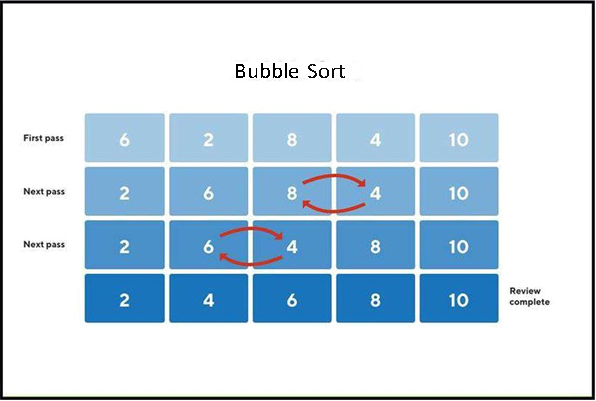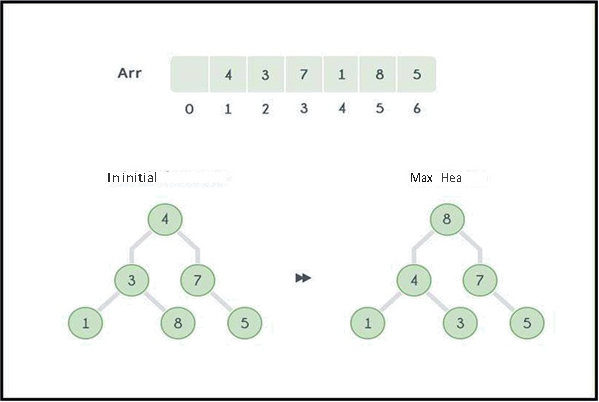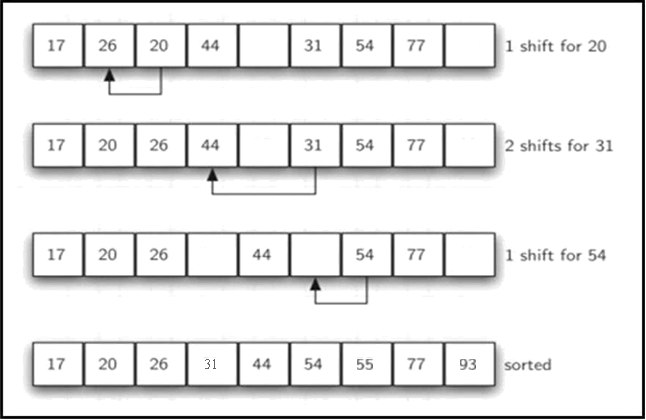## Bubble Sort

Bubble sort is the simplest sorting algorithm that works by repeatedly swapping the adjacent elements if they are in the wrong order. This algorithm is not suitable for large data sets as its average and worst-case time complexities are quite high.TypeScript Code:

function BubbleSort(arr: any[]){
let temp: any;
for (let i = 0; i < arr.length; i++) {
for (let j = 0; j < arr.length; j++) {
if (arr[i] < arr[j]){
temp = arr[i];
arr[i] = arr[j];
arr[j] = temp;
}

}
}
return arr;
}

let arr: number[] = [6, 2, 8, 4, 10];
console.log(BubbleSort(arr));

## Heap Sort

Heap sort is a comparison-based sorting technique based on the Binary Heap data structure. It is similar to the selection sort where we first find the minimum element and place the minimum element at the beginning. Repeat the same process for the remaining elements.TypeScript Code:

function swap(array: number[], index1: number, index2: number) {
[array[index1], array[index2]] = [array[index2], array[index1]];
}

// this is the equivalent to the heapify down method
function heapify(array: number[], index: number, length = array.length) {
let largest: number = index;
let left: number = index * 2 + 1;
let right: number = index * 2 + 2;

// compare element to it's left and right child
if (left < length && array[left] > array[largest]) {
largest = left;
}
if (right < length && array[right] > array[largest]) {
largest = right;
}

// if the parent node isn't the largest element, swap it with the largest child
if (largest !== index) {
swap(array, index, largest);

// continue to heapify down
heapify(array, largest, length);
}

return array;
}

function heapSort(array: number[]) {
// max heapify the array
for (let i = Math.floor(array.length / 2); i >= 0; i--) {
heapify(array, i)
}

// work backwards, moving max elements to the end of the array
for(let i = array.length - 1; i > 0; i--){
// max element of unsorted section of array is at index 0, swap with element at last index in unsorted array
swap(array, 0, i);

// re-heapify array, from beginning to the end of the unsorted section
heapify(array, 0, i);
}

return array;
}
let arr: number[] = [4, 3, 7, 1, 8, 5];
console.log(heapSort(arr));

## Insertion Sort

Insertion sort is a simple sorting algorithm that works similar to the way you sort playing cards in your hands The array is virtually split into a sorted and an unsorted part. Values from the unsorted part are picked and placed in the correct position in the sorted part.

function InsertionSort(arr: number[]){
let temp: number;
let j: number;
for (let i = 1; i < arr.length; i++){
temp = arr[i];
j = i - 1;
while (j >=0 && arr[j] > temp) {
arr[j + 1] = arr[j];
j--;
}
arr[j + 1] = temp;
}
return arr;
}
let arr: number[] = [54, 26, 93, 17, 77, 31, 44, 55, 20];
console.log(InsertionSort(arr));

## Merge Sort

Merge Sort is an algorithm that is considered an example of the divide and conquers strategy. So, in this algorithm, the array is initially divided into two equal halves and then they are combined in a sorted manner.

// First Step divide array into half
// Sort Each half
// Merge the result

// Dividing array into half and sorting each half
function sort(arr: number[]){
let middle: number = Math.floor(arr.length/2);
let left: number[] = new Array(middle);
let right: number[] = new Array(arr.length - middle);

// Base Condition
if (arr.length < 2){
return arr;
}
for (let i = 0; i < middle; i++) {
left[i] = arr[i];
}
for (let i = middle; i < arr.length; i++) {
right[i - middle] = arr[i];
}

// Sort Each Half.
sort(left);
sort(right);

return arr = merge(left, right, arr);
}

// Merge The result
function merge(left: number[], right: number[], result: number[]){
// i for iterate over left, j for iterate over right, k for iterate over result.
let i = 0, j = 0, k = 0;
while(i < left.length && j < right.length){
if (left[i] <= right[j]){
result[k++] = left[i++];
} else {
result[k++] = right[j++];
}
}
while (i < left.length){
result[k++] = left[i++];
}
while (j < right.length) {
result[k++] = right[j++];
}
return result;
}
let arr: number[] = [5, 2, 4, 7, 1, 3, 2, 6];
console.log(sort(arr));

## Quick Sort

Quick sort is also a divide-and-conquer algorithm. It picks an element as a pivot and partitions the given array around the picked pivot. This algorithm is used to quickly sort items within an array no matter how big the array is.

TypeScript Code:

function partition(array: number[], start: number, end: number) {
// For random pivot use => arr[Math.floor(Math.random() * (end - start + 1) + start)];
const pivotVal = array[Math.floor((start + end) / 2)];

while (start <= end) {
while (array[start] < pivotVal) {
start++;
}
while (array[end] > pivotVal) {
end--;
}

if (start <= end) {
// swap
let temp = array[start];
array[start] = array[end];
array[end] = temp;

start++;
end--;
}
}

return start;
}

function quickSort(array: number[], start: number = 0, end: number = arr.length - 1) {
if (start < end) {
const index = partition(array, start, end);

quickSort(array, start, index - 1);
quickSort(array, index, end);
}

return arr;
}

let arr: number[] = [7, -2, 4, 1, 6, 5, 0, -4, 2]
console.log(quickSort(arr));

## Selection Sort

The Selection sort algorithm sorts an array by repeatedly finding the minimum element (considering ascending order) from the unsorted part and putting it at the beginning.

TypeScript Code

function SelectionSort(arr: any[]){
let temp: any;
let jtrack: number = 0;
for (let i = 0; i < arr.length - 1; i++) {
jtrack = i;
for (let j = i + 1; j < arr.length; j++) {
if (arr[j] < arr[jtrack]){
jtrack = j;
}
}
// Swpping
if (jtrack != i){
temp = arr[i];
arr[i] = arr[jtrack];
arr[jtrack] = temp;
}
}
return arr;
}

let arr: number[] = [12, 10, 16, 11, 9, 7];
console.log(SelectionSort(arr));

Shell sort is mainly a variation of Insertion Sort. In insertion sort, we move elements only one position ahead. When an element has to be moved far ahead, many movements are involved. The idea of ShellSort is to allow the exchange of far items. In Shell sort, we make the array h-sorted for a large value of h. We keep reducing the value of h until it becomes 1. An array is said to be h-sorted if all sublists of every h’th element are sorted.TypeScript Code
class ShellSort {
array: number[] = [];
constructor(array: number[]) {
this.array = array;
}
sort(n: number) {
let gap = n;
while (gap > 0) {
for (let i = 0; i < this.array.length; i += 1) {
let unsortedIndex = i;
let tmp = this.array[i];
while ((unsortedIndex >= gap) && (this.array[unsortedIndex - gap] > tmp)) {
this.array[unsortedIndex] = this.array[unsortedIndex - gap];
unsortedIndex = unsortedIndex - gap;
}
this.array[unsortedIndex] = tmp;
}
if (Math.floor(gap / 2) !== 0) {
gap = Math.floor(gap / 2);
} else if (gap === 1) {
gap = 0;
} else {
gap = 1;
}
}
return this.array;
}
}

let arr: number[] = [17, 26, 20, 44, 55, 31, 54, 77, 93];
let shellSort: ShellSort = new ShellSort(arr);
console.log('====================================');
console.log(shellSort.sort(arr.length));
console.log('====================================');